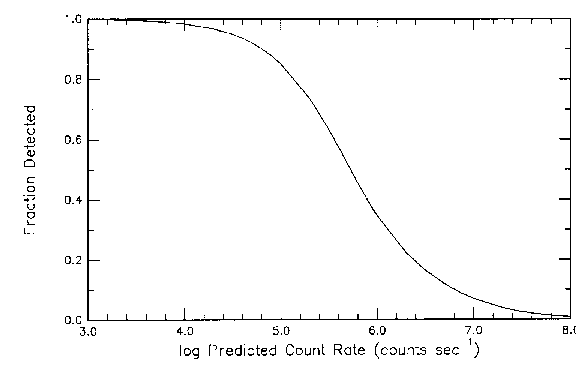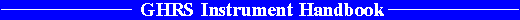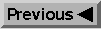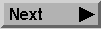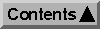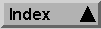Target Acquisition Reference Information

# Alternate Method for Predicting Target Acquisition Count Rates

Figure 7.1 on page 88 shows mean predicted count rates as a function of (B - V)0 color for the four acquisition mirrors. Also shown are the fits to the predicted count rates for different amounts of reddening. The label for each curve represents the color excess, E(B - V), applied to the spectra.

You can estimate target acquisition count rates using the plots instead of Table 7.1 on page 92:

• Determine the intrinsic color and magnitude of your object as well as its color excess.

• Read from the Figures (depending on the mirror used) the predicted count rate.

• Scale the predicted count rate by the ratio of apparent brightness of your object to an object with zero magnitude.

• Use Figure 7.2 on page 89 to correct for the "paired-pulse" effect.

## Two Examples

First, suppose you want to observe m Col, which has the following properties: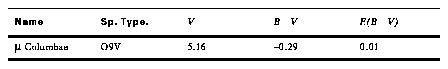Using the table, you would see that HR 8023, an O6 star with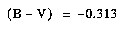is the closest match, giving a predicted count rate for the A2 mirror of 1.3 x 107counts s-1 for a V0 = 0 star. Multiplying this count rate by 10-0.45.13 gives 1.2 105 counts s-1. Reddening will decrease the counts slightly; calculation of the scale factor indicates that you should multiply by 0.93, giving a new count rate of 1.1 105 counts s-1. The dead-time correction factor estimates that only 83% of those counts will be detected, so one would expect approximately 94000 counts s-1 with this star. In fact, when m Col was observed early in Cycle 4, 19,600 counts were obtained in 0.2 seconds with the A2 mirror, which works out to 98,000 counts s-1, which is within 5% of the calculated value.

Second, consider a very red star such as Aldebaran: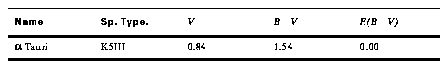Using the table, you would see that BD-1o3113 has a similar spectral type (K5III) and color (1.61). The calculated count rate for mirror A2 is then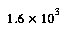counts per second. The adjustment for apparent magnitude is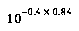, which yields a count rate of 530 per second, or 210 in 0.2 seconds. Early in Cycle 4 a Tau was acquired with mirror A2 and the count rate seen was 246 in 0.2 seconds, within 15% of the predicted value.

#### Figure 7.1: Mean target acquisition count rates for stars with the four mirrors of the GHRS. The numbers at the right indicate the appropriate E(B - V) for each curve.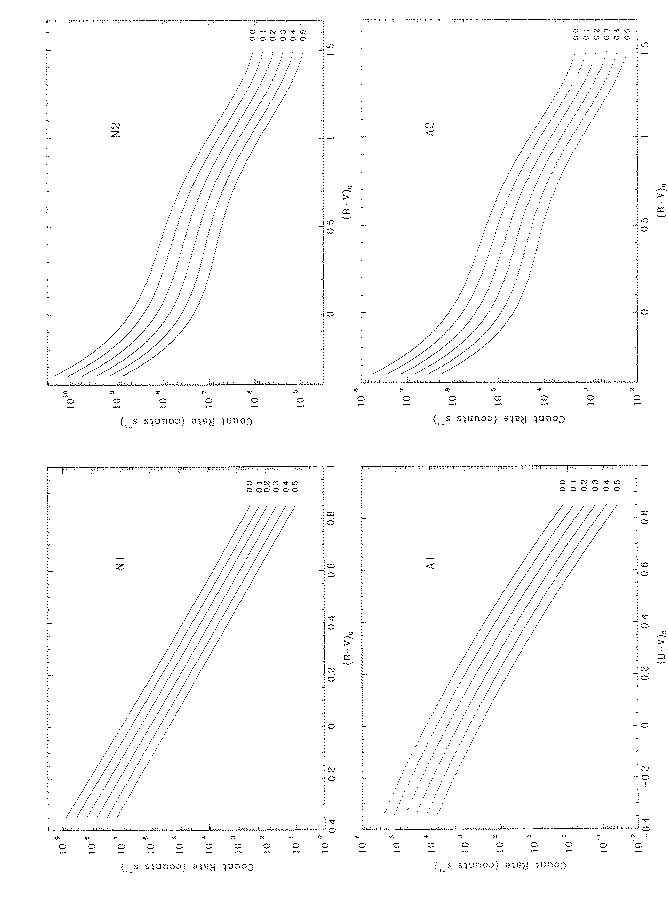#### Figure 7.2: Fraction of counts detected as a function of the true count rate, i.e., before the paired pulse correction.